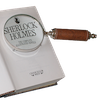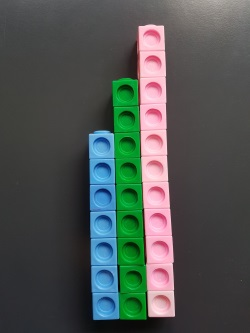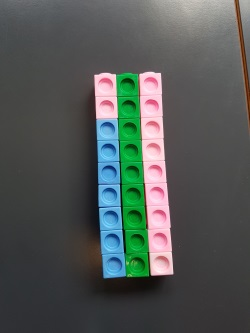#### You may also like### Number Detective

Follow the clues to find the mystery number.### Score

There are exactly 3 ways to add 4 odd numbers to get 10. Find all the ways of adding 8 odd numbers to get 20. To be sure of getting all the solutions you will need to be systematic. What about a total of 15 with 6 odd numbers?Great Granddad is very proud of his telegram from the Queen congratulating him on his hundredth birthday and he has friends who are even older than he is... When was he born?

### Why do this problem?

This low threshold high ceiling problem is rooted in a real-life context where number patterns naturally occur, thus appealing to students' curiosity about the world. Students can go through the process of playful exploration, noticing patterns, making conjectures, and finding ways to justify their conjectures. The justifications can be numerical, visual or even algebraic.

### Possible approach

This problem featured in an NRICH webinar in June 2019.

Show the image of the house numbers.
"What do you notice? What mathematical questions might you ask?" Give students a short time to think on their own, before talking to a partner and then sharing with the whole class.
Here are some examples of ideas that might emerge:

These look like house numbers
The houses might be next door neighbours
They are all odd
The next one might be 13
The total is 27

Tell the story: "These are the numbers I passed as I walked down the street. A bit later, I passed number 15, what do you think were the next two house numbers?" 17 and 19.
"I added together some sets of 3 house numbers together as I walked past. Your challenge is to explore the different totals that I might have made."

Give students some time to try out some examples by adding together sets of 3 consecutive odd numbers, and then bring the class together and collect their totals on the board. In order to perceive patterns, it's useful to write out the three numbers used, as well as the total.

"What do you notice about all the totals we have found?" (You may wish to write their noticings on the board.)
They are all odd
They are all multiples of 3
They are all 3 times the middle number
Once students have shared their noticings, invite them to choose one of them and talk with a partner about how they can explain, justify or prove it. Circulate and listen to their conversations, and choose some students who have different ways of explaining to feed back to the whole class.

Here are some possible representations that might emerge:

Using facts about odd and even numbers: When I add two odd numbers, the answer is even, so when I add three odd numbers the answer is even plus odd, which is odd. (This might be justified using a visual representation of odd and even numbers).

Numerical (using a generic example): 31 + 33 + 35, I take two off the 35 and add it to the 31 so I have three lots of 33. This will always work because the third number is two more and the first number is two less than the middle number.

Algebraic: I could write the three numbers as n, n+2 and n+4, so the total is 3n+6, which is 3(n+2). (Alternatively students could use n-2, n and n+2, giving a total of 3n.)

Visual:If I have towers to represent each consecutive odd number, then each tower will be two cubes higher than the last one. I can move two pink cubes and put them on top of the blue tower to make three towers the same height.

Next, you might like to ask the class to come up with other mathematically interesting questions about consecutive house numbers. Here are some that might emerge:
What would happen if I walked down the other side of the street instead (where all the houses have even numbers)?
What if I add together four house numbers instead of just three?
Or five house numbers? Or...

As before, invite students to explore one of their questions, making sure that they explain and justify any patterns they notice and conjectures they make.

### Key questions

What is the relationship between two consecutive odd numbers?
How could you represent the three house numbers? Using multilink/squared paper/algebra?
Can you see anything in your examples that would work in exactly the same way if you used three different consecutive odd numbers?
What's the same and what's different when you add three consecutive even numbers rather than three consecutive odd numbers?

### Possible support

Offer students some concrete representations of sets of three consecutive odd numbers, like the multilink representation above, or the equivalent picture on squared paper. Three Neighbours is structurally similar to this problem, but focuses on three consecutive numbers rather than three consecutive odd numbers.

### Possible extension

Students could explore which numbers it is not possible to make by adding together neighbouring house numbers (and for a challenging extension, prove any conjectures they make).

You could also challenge students by giving them a particular number and inviting them to work out how many different ways it can be made by adding together neighbouring house numbers. For example, 600 can be made in eleven different ways!

Summing Consecutive Numbers has some interesting extension questions to explore, based on a similar task looking at consecutive numbers rather than consecutive even and odd numbers.